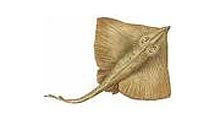jaymanntoday

Daily Fishing and Outdoor Report

Dogs love winter booties ... You better sleep with one eye open ...((((((((((((((((((((((((((((((((((((((((((((())))))))))))))))))))))))))))))))))))))))))))

Life lesson for kitten: No matter how hard you try you can't escape whatever this green thing is.Friday, February 20, 2015: The beach is fully hazardous to drive due to ice and snow below blown sand. It looks like an easy drive and in an instant you’re hopelessly bogged down. I'd advise staying off the beaches until Sunday rain is done.I hiked Holgate tip today. It might very well be the coldest I’ve ever hiked in my life. I got some truly weird looks at beaches swarmed over by stranded ice floes. Here’s a quick video look: http://www.youtube.com/watch?v=YV4fWTS_hkA&feature=youtu.be

Stu D. found an astounding bone on the beach in Holgate. It’s likely whale, but is mineralized, meaning it’s paleo. It weighs a solid 125 to 150 pounds. I have lifted an equally large modern whale bone – with one arm. This thing is impressively dense. It seems the Marine Mammal Stranding Center is interested in it. I sent them this video … a short vid by my standards but it was 15 degrees and honking winds. http://youtu.be/2r13qKMwHBg

Update: Update: MMSC supposes it is the jawbone of a baleen whale ... However, the weight is highly confusing since a ballen jawbone of that size shouldn't be more than 20 pounds.

((((((((((((((((((((((((((((((((((((()))))))))))))))))))))))))))))))))))))))))))

The ice floes out of BH and LE inlets is now many miles at sea, likely well into the shipping lanes. I alerted the USCG. While we aren't seeing much LBI-based boat traffic, everyday I see boats out there a ways. Some of these floes are so thick that could do hull damage.

(((((((((((((((((((((((((((((((((((())))))))))))))))))))))))))))))

"Don't worry. You can't blow this. There's just one thing you have to do ...((((((((((((((((((((((((((((((((((((((((((((((((((((())))))))))))))))))))))))))))))))))))))))))))))))

As we moan over the overpopulation of skate, on the other side of the pond they have become critically endangered, not only because of commercial fishing but also ravenous recreational fishing pressure.The common skate is the largest European flat fish, with females reaching lengths of 285cm and males 205cm. They are found in the north-east Atlantic from Madeira and northern Morocco to Iceland and northern Norway.

Common, long-nose, black and white skate are all endangered species. The common skate belies its name as it is becoming very rare in UK shallow seas and in European waters, and has been assessed as critically endangered by the IUCN.

(((((((((((((((((((((((((((((((((((((()))))))))))))))))))))))))))))))))))))))))))

Views: 395

Comment